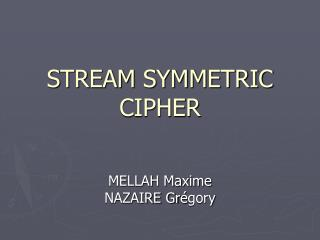DownloadDownload PresentationSTREAM SYMMETRIC CIPHER

# STREAM SYMMETRIC CIPHER

Télécharger la présentation## STREAM SYMMETRIC CIPHER

- - - - - - - - - - - - - - - - - - - - - - - - - - - E N D - - - - - - - - - - - - - - - - - - - - - - - - - - -
##### Presentation Transcript

1. STREAM SYMMETRIC CIPHER MELLAH MaximeNAZAIRE Grégory

2. DEFINITION • CIPHER : Language of cryptography-- There are many ciphers existing since coding messages has been a need for a long time, especially during war time-- The most ancient ciphers exist since Antiquities

3. DEFINITION • SYMMETRIC : Property of some ciphers-- When the same key is used to encrypt then decrypt a message then the cipher is called “symmetric” . -- On the contrary, ciphers are said to be asymmetric.

4. DEFINITION • STREAM : Property of some symmetric ciphers-- When a cipher treats the data digit by digit (usually bits or bytes), then it’s called“stream” (or state) ciphers-- Ciphers that are dealing with data flows (blocks of data) and much bigger data are called “block” ciphers.-- Stream ciphers are faster than block ciphers by a factor of 10 or even 100.

5. THE XOR OPERATION • When used twice, the XOR operation leads back to the original factor. • Here is a demonstration :(1*1)*1 = 0*1 = 1(1*0)*0 = 1*0 = 1 (0*1)*1 = 1*1 = 0 (0*0)*0 = 0*0 = 0

6. ONE-TIME PAD • 00101100.... 0101011Original plain text message • 01110111.... 1101011 Randomly generated key equal to message in length • 01011011.... 1000000 Encrypted message • 01110111.... 1101011 Key re-used to decrypt • 00101100.... 0101011 Original message restored